# Question:”Draw The Best Lewis Structure For CH2O. Based On The Lewis Structure For CH2O, Consider The Following. 1. The Electronic Geometry About The Carbon Atom Is 2. What Is The Strongest Intermolecular Force This Molecule Wo Exhibit? O London Dispersion (LDF) Dipole-dipole O Hydrogen Bonding 3. The Molecular Geometry About The Carbon Atom Is Select An Answer …”

“Draw The Best Lewis Structure For CH2O. Based On The Lewis Structure For CH2O, Consider The Following. 1. The Electronic Geometry About The Carbon Atom Is 2. What Is The Strongest Intermolecular Force This Molecule Wo Exhibit? O London Dispersion (LDF) Dipole-dipole O Hydrogen Bonding 3. The Molecular Geometry About The Carbon Atom Is Select An Answer …”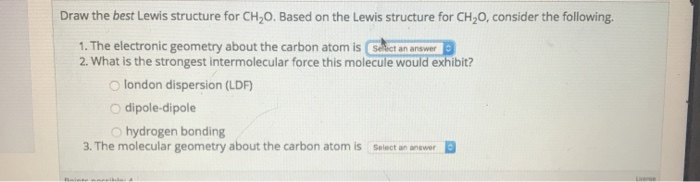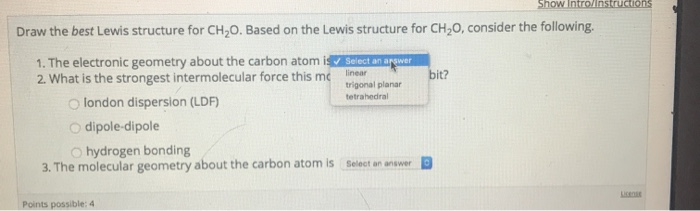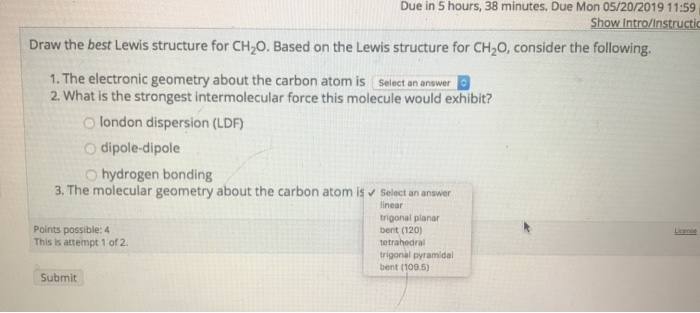Draw the best Lewis structure for CH2O. Based on the Lewis structure for CH2O, consider the following. 1. The electronic geometry about the carbon atom is 2. What is the strongest intermolecular force this molecule wo exhibit? o london dispersion (LDF) dipole-dipole O hydrogen bonding 3. The molecular geometry about the carbon atom is Select an answer
Draw the best Lewis structure for CH20. Based on the Lewis structure for CH20, consider the following 1. The electronic geometry about the carbon atom iSelect an a 2. What is the strongest intermolecular force this me unger O london dispersion (LDF) it? trigonal planar tetrahedral O dipole-dipole Ohydrogen bonding 3. The molecular geometry about the carbon atom is D Select an answer Points possible: 4
Due in 5 hours, 38 minutes. Due Mon 05/20/2019 11:59 Show Intro/Instructic Draw the best Lewis structure for CH2O. Based on the Lewis structure for CH2O, consider the following. 1. The electronic geometry about the carbon atom is (Select an answer 2. What is the strongest intermolecular force this molecule would exhibit? O london dispersion (LDF O dipole-dipole O hydrogen bonding 3. The molecular geometry about the carbon atom is Select an answer linear trigonal planar bent (120) Points possible: 4 This is attempt 1 of 2 tetrahedral trigonal pyramidal bent (109.5) Submit

Smart Teacher

ELECTRON DOMAIN GEOMETRY or electronic geometry

The lone pairs on the molecule are counted as electron domain (E.D.). For changing shape, only lone pairs of electrons and primary bonds between atoms are included. The “extra” bonds of double and triple bonds don’t count. The number of electron domains is the primary factor in determining the shape of a molecule. This starts by figuring out the electron domain geometry.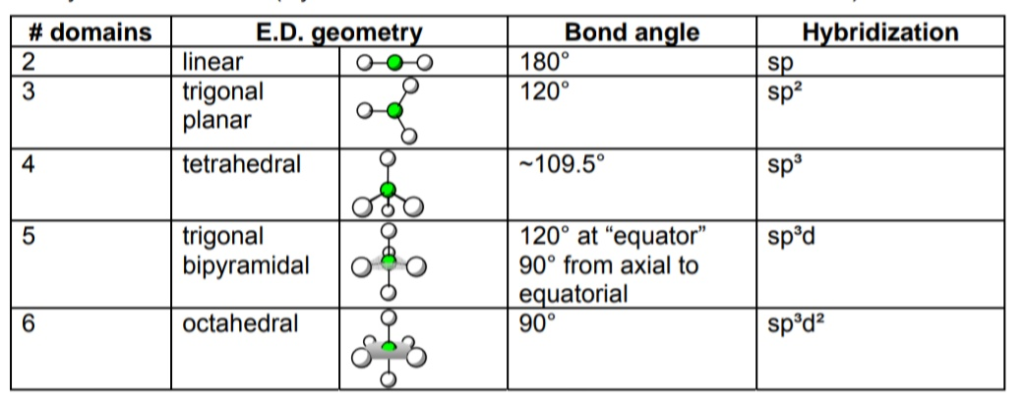MOLECULAR GEOMETRY

molecular geometry around a central atom is the shape of the actual bonds with the other atoms. To determine the molecular geometry for a particular atom, knowledge of electron domain geometry and the number of lone pairs around the central atom need to be known. The table below shows the progression of changes to the geometry when atoms are replaced by lone pairs.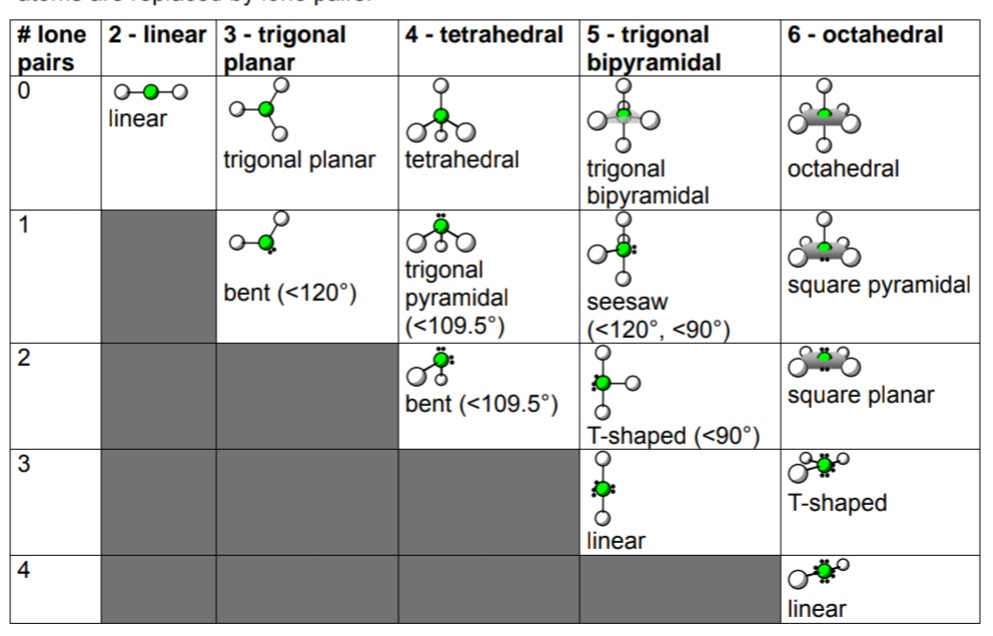The Lewis structure of CH2O is given below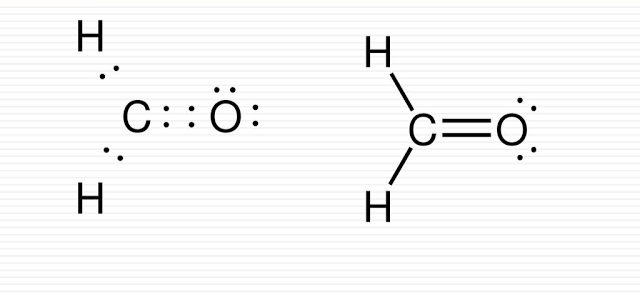There are 4 valence electrons in carbon, 1 each in hydrogen and 6 in oxygen, so there are 12 electrons total. After bonding carbon to
the other atoms, there are 6 left. The oxygen atom needs 6 more to get its octet, but that leave the central atom short, so a double bond between oxygen and carbon is created. The Lewis structure is given above.
There are three electron domains here, not four. (The extra bond in the double bond isn’t an electron domain.) The E.D. geometry is trigonal planar, and because there are no lone pairs, the molecular geometry is also trigonal planar.

INTERMOLECULAR FORCES IN CH​​​​​2​​​​O

CH2O is a covalent molecule as the electrons are shared in between atoms. So,this is not ionic or there is no ionic interaction in between the molecule.

CH2O doesn’t contain any of the H—O, H—N,H—F bond which is necessary for making hydrogen bond. So,there is no hydrogen bonding interaction between the molecule.

Due to the electronegativity difference between the O and C atom in CH2O dipole character arises in >C=O bond which makes the molecule polar. So, there exists dipole-dipole interactions.

And London dispersion forces are present in all type of compounds and so as in CH2O.

Dipole interactions are stronger than London dispersion forces.So,the predominant intermolecular forces in CH2O is dipole-dipole interactions.

Answers: 1. Trigonal planar, 2. Dipole-dipole, 3. Trigonal planar